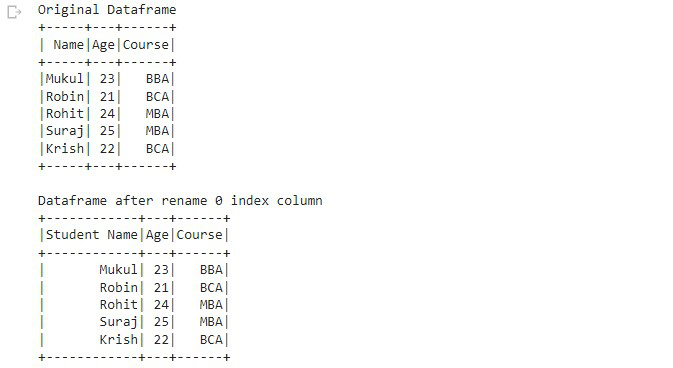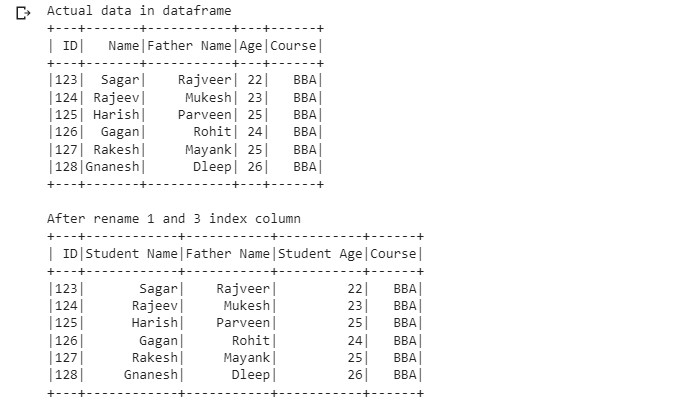# How to rename a PySpark dataframe column by index?

• Last Updated : 28 Mar, 2022

In this article, we are going to know how to rename a PySpark Dataframe column by index using Python. we can rename columns by index using Dataframe.withColumnRenamed() and Dataframe.columns[] methods.  with the help of Dataframe.columns[] we get the name of the column on the particular index and then we replace this name with another name using the withColumnRenamed() method.

Example 1: The following program is to rename a column by its index.

## Python3

 `# importing required module``import` `pyspark``from` `pyspark.sql ``import` `SparkSession`` ` `# creating sparksession and giving``spark ``=` `SparkSession.builder.appName(``'sparkdf'``).getOrCreate()`` ` `# demo data of college students``data ``=` `[[``"Mukul"``, ``23``, ``"BBA"``],``        ``[``"Robin"``, ``21``, ``"BCA"``],``        ``[``"Rohit"``, ``24``, ``"MBA"``],``        ``[``"Suraj"``, ``25``, ``"MBA"``],``        ``[``"Krish"``, ``22``, ``"BCA"``]]`` ` `# giving column names of dataframe``columns ``=` `[``"Name"``, ``"Age"``, ``"Course"``]`` ` `# creating a dataframe``dataframe ``=` `spark.createDataFrame(data, columns)`` ` `# Rename dataframe``df ``=` `dataframe.withColumnRenamed(dataframe.columns[``0``],``                                 ``"Student Name"``)`` ` `# Original dataframe``print``(``"Original Dataframe"``)``dataframe.show()`` ` `# Dataframe after rename column``print``(``"Dataframe after rename 0 index column"``)``df.show()`

Output:Example 2: The following program is to rename multiple columns by these indexes.

## Python3

 `# importing module``import` `pyspark`` ` `# importing sparksession from``# pyspark.sql module``from` `pyspark.sql ``import` `SparkSession`` ` `# creating sparksession and giving``# an app name``spark ``=` `SparkSession.builder.appName(``'sparkdf'``).getOrCreate()`` ` `# list  of students  data``data ``=` `[[``123``, ``"Sagar"``,   ``"Rajveer"``, ``22``, ``"BBA"``],``        ``[``124``, ``"Rajeev"``,  ``"Mukesh"``,  ``23``, ``"BBA"``],``        ``[``125``, ``"Harish"``,  ``"Parveen"``, ``25``, ``"BBA"``],``        ``[``126``, ``"Gagan"``,   ``"Rohit"``,   ``24``, ``"BBA"``],``        ``[``127``, ``"Rakesh"``,  ``"Mayank"``,  ``25``, ``"BBA"``],``        ``[``128``, ``"Gnanesh"``, ``"Dleep"``,   ``26``, ``"BBA"``]]`` ` `# specify column names``columns ``=` `[``'ID'``, ``'Name'``, ``'Father Name'``,``           ``'Age'``, ``"Course"``, ]`` ` `# creating a dataframe from the lists of data``dataframe ``=` `spark.createDataFrame(data, columns)`` ` `# display original dataframe``print``(``'Actual data in dataframe'``)``dataframe.show()`` ` `# Rename column``df ``=` `dataframe.withColumnRenamed(dataframe.columns[``1``], ``                                 ``"Student Name"``).withColumnRenamed(``    ``dataframe.columns[``3``], ``"Student Age"``)`` ` `# display dataframe after rename column``print``(``'After rename 1 and 3 index column'``)``df.show()`

Output:My Personal Notes arrow_drop_up